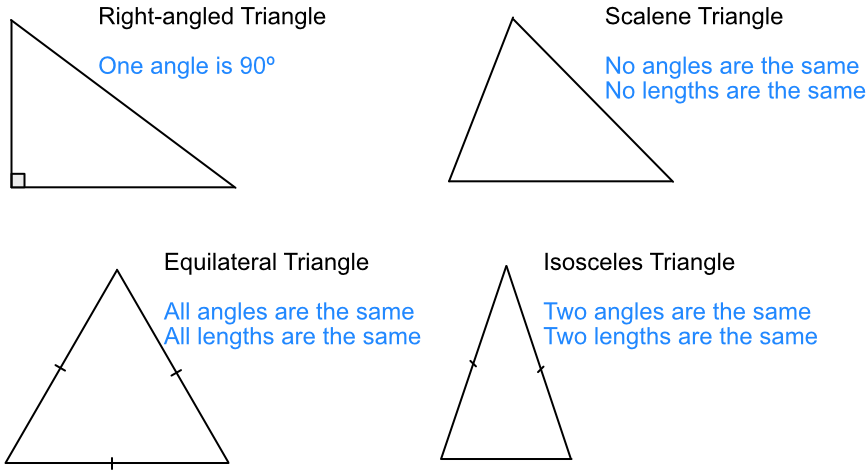Properties of Triangles

## Properties of Triangles

There are four types of triangle:(A mark on a line means that it is the same length as another line with the same mark).

## Example 1

In a triangle, one angle is 30º and a second angle is 60º.

What type of triangle is it?

The two angles add up to 90º.

There are 180º in a triangle, so the remaining angle is 90º.

A triangle has sides of length x, x and 2x.